• ## sobel

2021-05-13 11:03:49
Sobel算法代码（python） import numpy as np import imageio #先获取灰度图 def rgb2gray(rgb): “”" convert rgb image into gray Args: rgb: grb image with numpy type Returns: gray: gray image “”" gray =...
Sobel算法代码（python）
import numpy as np
import imageio
#先获取灰度图
def rgb2gray(rgb):
“”"
convert rgb image into gray
Args:
rgb: grb image with numpy type
Returns:
gray: gray image
“”"
gray = rgb[:, :, 0] * 0.299 + rgb[:, :, 1] * 0.587 + rgb[:, :, 2] * 0.114
return gray
gray = rgb2gray(image_in)
imageio.imsave(im=gray.astype(np.uint8), uri=“gray_image.jpg”)
#然后进行sobel操作
def sobel(image):
“”"
implement sobel compute on a single gray image
Args:
image:  a gray image
Returns:
the image that have been processd by sobel
Raises:
ValueError: the input's shape image must bigger than sobel(3x3)
"""
# change the data type of input image into np.uint8(cheaper compute cost)
image = image.astype(np.uint8)
assert len(image.shape) == 2
if image.shape < 3 or image.shape < 3:
raise ValueError("The width and height of input image must bigger than 3")
# create temp image container
sobel_image_x = np.zeros(shape=image.shape)
sobel_image_y = np.zeros(shape=image.shape)
# create sobel
sobel_x = np.array([[-1, 0, 1], [-2, 0, 2], [-1, 0, 1]])
sobel_y = np.array([[-1, -2, -1], [0, 0, 0], [1, 2, 1]])

for i in range(image.shape-2):
for j in range(image.shape-2):
# have to use np.abs() to make sure the value is positive
sobel_image_x[i, j] = np.abs(np.sum(image[i:i+3, j:j+3] * sobel_x))
sobel_image_y[i, j] = np.abs(np.sum(image[i:i+3, j:j+3] * sobel_y))
sobel_image = np.sqrt(sobel_image_x*sobel_image_x + sobel_image_y*sobel_image_y).astype(np.uint8)

return sobel_image

rgb = rgb2gray(image_in)
sobel_image = sobel(rgb)
imageio.imsave(im=sobel_image, uri=“sobel_image.jpg”)


展开全文计算机视觉
• sobel滤波器介绍sobel滤波器常用来提取灰度图像的水平边缘(水平特征)和竖直边缘(竖直特征)二. sobel算子纵向算子，提取图像水平边缘 ↑横向算子，提取图像竖直边缘 ↑三. 实验：python实现sobel算子并将算子作用于...
一. sobel滤波器介绍sobel滤波器常用来提取灰度图像的水平边缘(水平特征)和竖直边缘(竖直特征)二. sobel算子纵向算子，提取图像水平边缘 ↑横向算子，提取图像竖直边缘 ↑三. 实验：python实现sobel算子并将算子作用于图像import cv2import numpy as np# Gray scaledef BGR2GRAY(img):b = img[:, :, 0].copy()g = img[:, :, 1].copy()r = img[:, :, 2].copy()# Gray scaleout = 0.2126 * r + 0.7152 * g + 0.0722 * bout = out.astype(np.uint8)return out# sobel filterdef sobel_filter(img, K_size=3):if len(img.shape) == 3:H, W, C = img.shapeelse:H, W = img.shape# Zero paddingpad = K_size // 2out = np.zeros((H + pad * 2, W + pad * 2), dtype=np.float)out[pad: pad + H, pad: pad + W] = img.copy().astype(np.float)tmp = out.copy()out_v = out.copy()out_h = out.copy()## Sobel verticalKv = [[1., 2., 1.],[0., 0., 0.], [-1., -2., -1.]]## Sobel horizontalKh = [[1., 0., -1.],[2., 0., -2.],[1., 0., -1.]]# filteringfor y in range(H):for x in range(W):out_v[pad + y, pad + x] = np.sum(Kv * (tmp[y: y + K_size, x: x + K_size]))out_h[pad + y, pad + x] = np.sum(Kh * (tmp[y: y + K_size, x: x + K_size]))out_v = np.clip(out_v, 0, 255)out_h = np.clip(out_h, 0, 255)out_v = out_v[pad: pad + H, pad: pad + W].astype(np.uint8)out_h = out_h[pad: pad + H, pad: pad + W].astype(np.uint8)return out_v, out_h# Read imageimg = cv2.imread("../paojie.jpg").astype(np.float)# grayscalegray = BGR2GRAY(img)# sobel filteringout_v, out_h = sobel_filter(gray, K_size=3)# Save resultcv2.imwrite("out_g.jpg",gray)cv2.imshow("result_g",gray)cv2.imwrite("out_v.jpg", out_v)cv2.imshow("result_v", out_v)cv2.imwrite("out_h.jpg", out_h)cv2.imshow("result_h", out_h)cv2.waitKey(0)cv2.destroyAllWindows()四. 实验结果原图 ↑原图转换为灰度图像 ↑sobel横向算子提取了图像的竖直特征 ↑sobel纵向算子提取了图像的水平特征 ↑从本实验结果我们观察到，在提取图像在水平或者垂直方向上的线条或轮廓时，可以使用sobel算子。五. 参考内容：
展开全文• <div><p>the sobel operator is returning and image with a Uint8ClampedArray in the return statement, it should return a Float32Array image for the derivatives, no? and the convolutions made inside the ...
• OpenCV 2.4 C++ 边缘梯度计算 --- sobel 使用说明 Sobel算子 Sobel 算子是一个离散微分算子 (discrete differentiation ope...

https://docs.opencv.org/2.4/doc/tutorials/imgproc/imgtrans/sobel_derivatives/sobel_derivatives.html
OpenCV 2.4 C++ 边缘梯度计算 --- sobel 使用说明
Sobel算子
Sobel 算子是一个离散微分算子 (discrete differentiation operator)。 它结合了高斯平滑和微分求导，用来计算图像灰度函数的近似梯度。
一.基础知识介绍
图像的边缘
图像的边缘从数学上是如何表示的呢？
图像的边缘上，邻近的像素值应当显著地改变了。而在数学上，导数是表示改变快慢的一种方法，一个函数在某一点的导数描述了这个函数在这一点附近的变化率。
梯度可谓是多元函数的偏导，表示某一函数在该点处的方向导数沿着该方向取得最大值，即函数在该点处沿着该方向（此梯度的方向）变化最快，变化率最大（为该梯度的模）。。
用更加形象的图像来解释,假设我们有一张一维图形。下图中灰度值的“跃升”表示边缘的存在：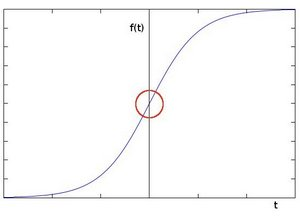使用一阶微分求导我们可以更加清晰的看到边缘“跃升”的存在(这里显示为高峰值)：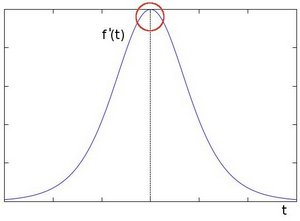由此我们可以得出：边缘可以通过定位梯度值大于邻域的相素的方法找到。
卷积
卷积可以近似地表示求导运算。
那么卷积是什么呢？

卷积是在每一个图像块与某个算子（核）之间进行的运算。

核呢？

核就是一个固定大小的数值数组。该数组带有一个锚点 ，一般位于数组中央。

可是这怎么运算啊？

假如你想得到图像的某个特定位置的卷积值，可用下列方法计算：
将核的锚点放在该特定位置的像素上，同时，核内的其他值与该像素邻域的各像素重合；
将核内各值与相应像素值相乘，并将乘积相加；
将所得结果放到与锚点对应的像素上；
对图像所有像素重复上述过程。
用公式表示上述过程如下：

在图像边缘的卷积怎么办呢？

计算卷积前，OpenCV通过复制源图像的边界创建虚拟像素，这样边缘的地方也有足够像素计算卷积了。

二.Sobel的卷积实现
Sobel是采用卷积的计算方法实现的。假设被作用的图像为,在两个方向上求导：
水平变化求导：将与一个奇数大小的内核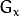进行卷积。比如，当内核大小为3时,的计算结果为图1
垂直变化求导：将 I 与一个奇数大小的内核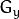进行卷积。比如，当内核大小为3时,的计算结果为图2
在图像的每一点，结合以上两个结果求出近似梯度大小 ，如图3
计算梯度方向，如图4   （如果以上的角度Θ等于零，即代表图像该处拥有纵向边缘，左方较右方暗）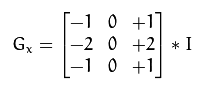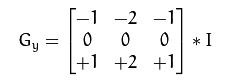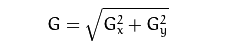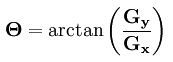图1                                  图2                                    图3                          图4
三.Code

先来看一下C++下 Sobel 的定义

cv：Sobel(  InputArray src ,  OutputArray dst,  int ddepth,  int dx,  int dy,  int ksize=3,

double scale=1,double delta=0,intborderType=BORDER_DEFAULT )

各参数的意义如下：

src – 输入图像。dst – 输出图像，与输入图像同样大小，拥有同样个数的通道。
ddepth –输出图片深度；下面是输入图像支持深度和输出图像支持深度的关系：

src.depth() = CV_8U, ddepth = -1/CV_16S/CV_32F/CV_64F

src.depth() = CV_16U/CV_16S, ddepth = -1/CV_32F/CV_64F

src.depth() = CV_32F, ddepth = -1/CV_32F/CV_64F

src.depth() = CV_64F, ddepth = -1/CV_64F

当 ddepth为-1时， 输出图像将和输入图像有相同的深度。输入8位图像则会截取顶端的导数。

xorder – x方向导数运算参数。yorder – y方向导数运算参数。
ksize – Sobel内核的大小，可以是：1，3，5，7。  注意：只可以是小于7 的奇数
scale – 可选的缩放导数的比例常数。delta – 可选的增量常数被叠加到导数中。borderType – 用于判断图像边界的模式。

#include "opencv2/imgproc/imgproc.hpp"
#include "opencv2/highgui/highgui.hpp"
#include <stdlib.h>
#include <stdio.h>

using namespace cv;

/** @function main */
int main( int argc, char** argv )
{

Mat src, src_gray;
char* window_name = "Sobel Demo - Simple Edge Detector";
int scale = 1;
int delta = 0;
int ddepth = CV_16S;

int c;

if( !src.data )
{ return -1; }
///高斯模糊---apply a GaussianBlur to our image to reduce the noise ( kernel size = 3 )
GaussianBlur( src, src, Size(3,3), 0, 0, BORDER_DEFAULT );

/// Convert it to gray
cvtColor( src, src_gray, CV_BGR2GRAY );

/// Create window
namedWindow( window_name, CV_WINDOW_AUTOSIZE );
///calculate the “derivatives” in x and y directions
//Scharr( src_gray, grad_x, ddepth, 1, 0, scale, delta, BORDER_DEFAULT );
Sobel( src_gray, grad_x, ddepth, 1, 0, 3, scale, delta, BORDER_DEFAULT );
//Scharr( src_gray, grad_y, ddepth, 0, 1, scale, delta, BORDER_DEFAULT );
Sobel( src_gray, grad_y, ddepth, 0, 1, 3, scale, delta, BORDER_DEFAULT );

/// Total Gradient (approximate近似)---we try to approximate the gradient by adding both directional gradients      (note that this is not an exact calculation at all! but it is good for our purposes).

waitKey(0);

return 0;
}

转载于:https://www.cnblogs.com/Jessica-jie/p/8531177.html
展开全文• sobel 算法，详细介绍如何使用FIFO 实现sobel算法对图像进行处理
• 彻底理解数字图像处理中的卷积-以Sobel算子为例https://www.cnblogs.com/freeblues/p/5738987.html python自编程序实现——robert算子、sobel算子、Laplace算子进行图像边缘提取...


彻底理解数字图像处理中的卷积-以Sobel算子为例https://www.cnblogs.com/freeblues/p/5738987.html

python自编程序实现——robert算子、sobel算子、Laplace算子进行图像边缘提取https://www.cnblogs.com/zhanghaiyan/p/9902057.html

基于sobel算子的边缘检测 (python实现)https://blog.csdn.net/weixin_42731543/article/details/103948583

sobel算子原理与实现https://blog.csdn.net/qq_37124237/article/details/82183177

展开全文• halcon sobel 边缘检测 sobel_dir sobel_dir - 使用Sobel算子检测边缘（振幅和方向）。 sobel_dir(Image : EdgeAmplitude, EdgeDirection : FilterType, Size : )
• c++ opencv中的sobel算子用例，Sobel算子是像素图像边缘检测中最重要的算子之一，在机器学习、数字媒体、计算机视觉等信息科技领域起着举足轻重的作用。
• ## Sobel算子及cvSobel

千次阅读 2011-03-17 17:10:00扩展
• Sobel算子 卷积的应用 图像边缘提取 边缘：是像素值发生跃迁的地方，是图像的显著特征之一，在图像特的显著特征之一，在图像特诊提取、对象检测、模式识别等方面都有重要的作用。 捕捉/提取边缘：对图像求它的一阶...opencv c++
• Sobel 导数 目标 本文档尝试解答如下问题: 如何使用OpenCV函数Sobel对图像求导。 如何使用OpenCV函数Scharr更准确地计算核的导数。 原理 Note 以下内容来自于Bradski和Kaehler的大作：...
• FPGA SOBEL运算 具体解释参考http://blog.csdn.net/yunge812/article/details/79649970
• 1.2.1. Sobel算法分析索贝尔算子(Sobeloperator)主要用作边缘检测，在技术上，它是一离散性差分算子，用来运算图像亮度函数的灰度之近似值。在图像的任何一点使用此算子，将会产生对应的灰度矢量或是其法矢量。Sobel...
• 这里给大家分享一个Sobel算子matlab实现的源代码。
• 本文介绍的是常用图像边缘检测算法 ——Sobel边缘检测算法。
• <div><p>The sobel filter in the Data Transforms menu does not do what a user would want. Scipy's sobel is only a 1D filter, but most image analysis should be done in 2D. See attached python script...
• ## sobel算法

千次阅读 2017-04-03 15:05:39
本文代码来自sobel导数，该网站对opencv上图像处理的算法说明都很详细。如下： Sobel 导数 目标 本文档尝试解答如下问题: 如何使用OpenCV函数 Sobel 对图像求导。如何使用OpenCV函数 Scharr 更...
• 最近看了一些Sobel算法，并试了一下，源码如下： private void Sobel(Bitmap img) { int width = img.Width; int height = img.Height; int[,] Gx = new int[3, 3]{ {-1, 0, 1 }, ......